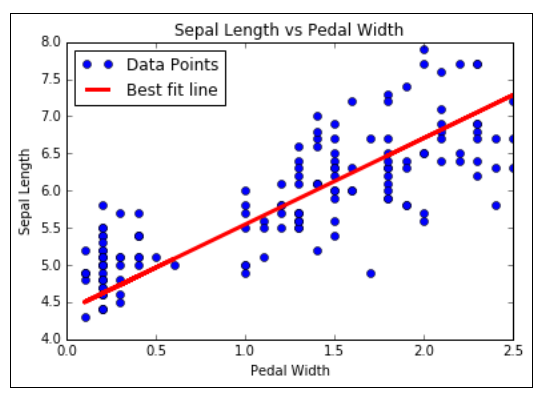# Learning The TensorFlow Way of Linear RegressionWe will loop through batches of data points and let TensorFlow update the slope and y-intercept. Instead of generated data, we will use the iris dataset that is built into the Scikit Learn. Specifically, we will find an optimal line through data points where the x-value is the petal width and the y-value is the sepal length. We choose these two because there appears to be a linear relationship between them, as we will see in the graphs at the end. We will also talk more about the effects of different loss functions in the next section, but for now we will use the L2 loss function. Learning The TensorFlow Way of Linear Regression

We will loop through batches of data points and let TensorFlow update the slope and y-intercept. Instead of generated data, we will use the iris dataset that is built into the Scikit Learn. Specifically, we will find an optimal line through data points where the x-value is the petal width and the y-value is the sepal length. We choose these two because there appears to be a linear relationship between them, as we will see in the graphs at the end. We will also talk more about the effects of different loss functions in the next section, but for now we will use the L2 loss function.

### How to do it…

``````import matplotlib.pyplot as plt
import numpy as np
import tensorflow as tf

from sklearn import datasets
from tensorflow.python.framework import ops
ops.reset_default_graph()
sess = tf.Session()
x_vals = np.array([x for x in iris.data])
y_vals = np.array([y for y in iris.data])``````

2. We then declare our learning rate, batch size, placeholders, and model variables:

``````learning_rate = 0.05
batch_size = 25

x_data = tf.placeholder(shape=[None, 1], dtype=tf.float32)
y_target = tf.placeholder(shape=[None, 1], dtype=tf.float32)
A = tf.Variable(tf.random_normal(shape=[1,1]))
b = tf.Variable(tf.random_normal(shape=[1,1]))``````

## Most popular Data Science and Machine Learning courses — July 2020

Most popular Data Science and Machine Learning courses — August 2020. This list was last updated in August 2020 — and will be updated regularly so as to keep it relevant

## Artificial Neural Network | Deep Learning with TensorFlow and Artificial Intelligence

Artificial Neural Network | Deep Learning with Tensorflow and Artificial Intelligence | I have talked about Artificial neural networks and its implementation in TensorFlow using google colab. You will learn: What is an Artificial Neural Network? Building your neural network using Tensorflow.

## Artificial Intelligence vs Machine Learning vs Data Science

Artificial Intelligence, Machine Learning, and Data Science are amongst a few terms that have become extremely popular amongst professionals in almost all the fields.

## Convolutional Neural Network | Deep Learning with TensorFlow and Artificial Intelligence

Convolutional Neural Network | Deep Learning with Tensorflow and Artificial Intelligence | I have talked about Convolutional neural networks and their implementation in TensorFlow using Google Colab. You will learn: What is a Convolutional Neural Network? Four different layers of CNNs; Building your CNNs using Tensorflow.

## AI(Artificial Intelligence): The Business Benefits of Machine Learning

Enroll now at CETPA, the best Institute in India for Artificial Intelligence Online Training Course and Certification for students & working professionals & avail 50% instant discount.